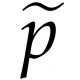8:18 AM
0Let $f(n)=(1 + (-1)^{1 + n})/2$ (which gives 1, 0, 1, 0 ... as n increases from 1 on) and $\lambda(n)$ be the Liouville lambda function. Can I compute a Dirichlet series for $f(n)\lambda(n)$? I could not find it in the Gould and Shonhiwa catalog of known Dirichlet series, but I am convinced it mu...Dirichlet convolution
In mathematics, the Dirichlet convolution is a binary operation defined for arithmetic functions; it is important in number theory. It was developed by Peter Gustav Lejeune Dirichlet. == Definition == If f , g : N → C {\displaystyle f,g:\mathbb {N} \to \mathbb {C} } are two arithmetic functions from the positive integers to the complex numbers, the Dirichlet convolution f ∗ g is a new arithmetic function defined by: ( f ∗...

6 hours later…2:35 PM
A question with a deprecated tag was bumped - what would be a good replacement for ? Bounding the entropy of a convolution
9Say we have a function $f:\mathbb{Z}_2^n \to \mathbb{R}$, such that $\sum _{x\in \mathbb{Z}_2^n} f(x)^2 = 1$ (so we can think of $\{ f(x)^2\} _{x\in \mathbb{Z}_2^n}$ as a distribution). It is natural to define the entropy of such function $f$ as follows: H(f) = -\sum _{x \in \mathbb{Z}_2^n} f(x...

2 hours later…4:56 PM
7The example I first learned was the following: a 2-D TQFT is equivalent to a Frobenius algebra. This is discussed and stated as a folk theorem by Voronov; later, a careful proof was written up and published by Lowell Abrams. See also the book by Joachim Kock.

The post contains this link: xxx.lanl.gov/abs/hep-th/9401023 - does somebody know how can the xxx.lanl.gov links be replaced to get working links. I do not see this domain listed here: Domains with dead links.
14In this post, Justin gives a quote about Raoul Bott that has this line in it: He talked about 'folk' theorems... theorems everyone knew, but were never written down. What are some good/interesting examples of these types of theorems?

A temporary comment - to update the list of linked posts: Folk Functorial Figuring. — Martin Sleziak 1 min ago
5In category theory there is a 'folk' model structure on the category Cat, where the weak equivalences are the equivalences of categories. There is a similar model structure on 2Cat, with weak equivalences being equivalences of 2-categories (weak ones, I presume) The former was not written down fo...

@theHigherGeometer The question "Examples of "folk theorems"" was recently bumped. I see that there is a dead link in your answer.
> Andre Joyal is not in favour of the name 'folk model structure', and there was discussion on this at the nForum (starting at that comment and continuing). That the existence of this model structure is a 'folk' theorem is a bit of folklore itself, as pointed out by Joyal at this comment.
I do not see anything in the Wayback Machine.
Maybe there is something at this link: nforum.ncatlab.org/discussion/649 ?
But since it is your post, I think it's better if I leave editing to you.